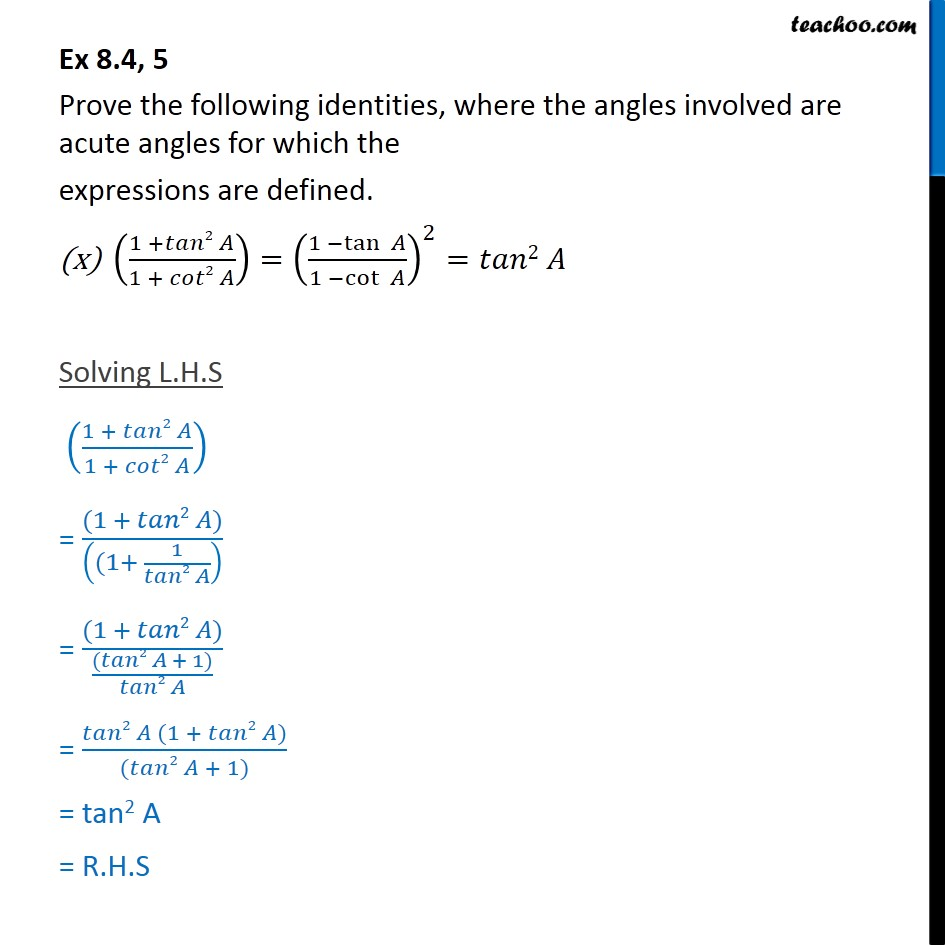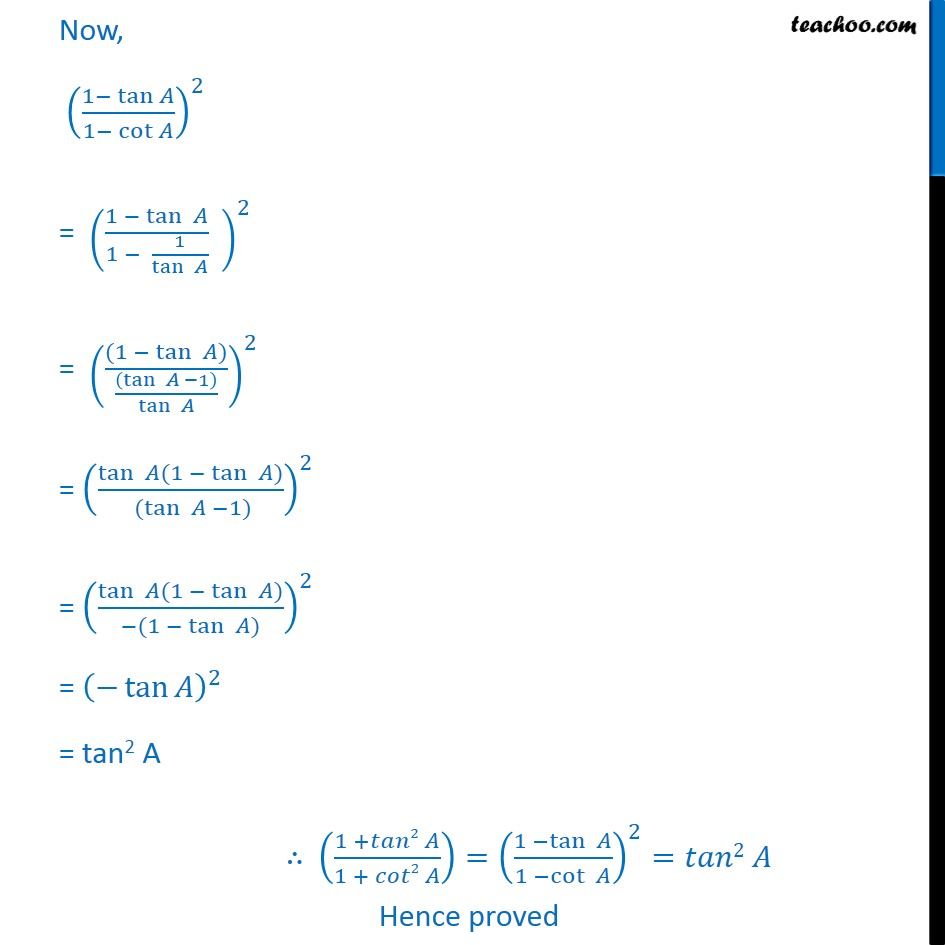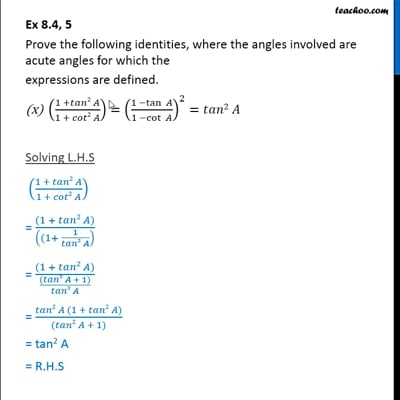Ex 8.4

Chapter 8 Class 10 Introduction to Trignometry
Serial order wiseThis video is only available for Teachoo black users

Maths Crash Course - Live lectures + all videos + Real time Doubt solving!

### Transcript

Ex 8.4, 5 Prove the following identities, where the angles involved are acute angles for which the expressions are defined. ((1 + 2 )/(1 + 2 ))=((1 tan )/(1 cot ))^2= 2 Solving L.H.S ((1 + 2 )/(1 + 2 )) = ((1 + 2 ))/(((1+ 1/( 2 )) ) = ((1 + 2 ))/((( 2 + 1))/( 2 )) = ( 2 (1 + 2 ))/(( 2 + 1)) = tan2 A = R.H.S Now, ((1 tan )/(1 cot ))^2 = ((1 tan )/(1 1/tan ) " " )^2 = (((1 tan ) )/(((tan 1 ))/tan ))^2 = (tan (1 tan ) /( (tan 1) ))^2 = (tan (1 tan ) /( (1 tan ) ))^2 = ( tan )^2 = tan2 A